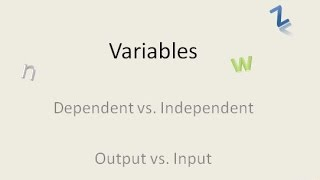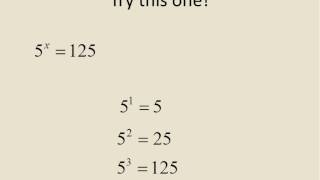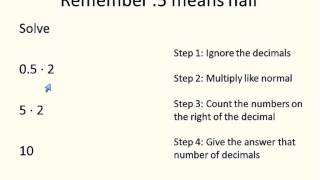## Eric BuffingtonEric Buffington Teacher United States of America

## User videosDependent vs Independent Variables
Have you ever struggled knowing if the variable was dependent or independent. This lesson will look at examples to give an explanation about dependent vs independent variables. I think it is safe to say you will be... independent.Introduction to Solving Exponents with Variables
This video is an introduction to solving positive and negative exponent equations. It shows some short cuts, and this lesson will look at examples to give an explanation about how exponents work.Multiplying with Decimals
This lesson will look at examples to give an explanation about multiplying numbers that have decimals.Square Roots with Variables - New
This lesson will look at examples to give an explanation about how to find the square root of a term that has variables and numbers.Multiplying Integers
Have you ever struggled with rules for multiplying with negative numbers? This video shows some great examples and tips that will help explain how to multiply integers.